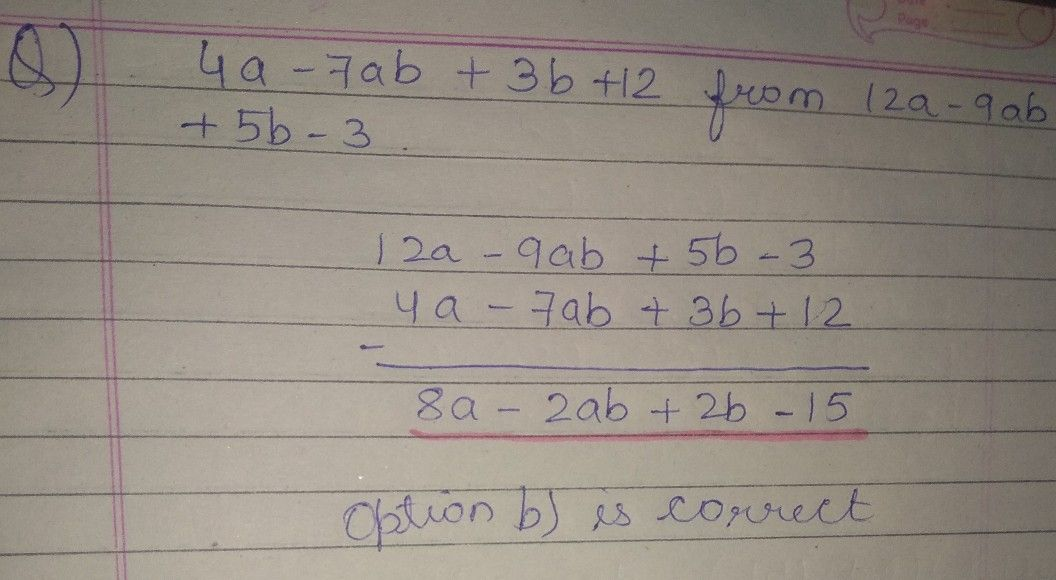Symbol
Problem$22.$ If we subtract 4a $-7ab+3b+12$ from $12a$ $-9ab+5b-3$ then we get $○$ O (a) $8a+2ab+2b+15$ $○$ $\left(b\right)$ $8a$ $-2ab+2b-15$ $○$ $\left(c\right)$ $8a-2ab+2b-15$ $○$ (d) $8a-2ab-2b-15$ $○$ $\left($ $e\right)$ None
7th-9th grade
Other
SolutionQanda teacher - ruqaiya56